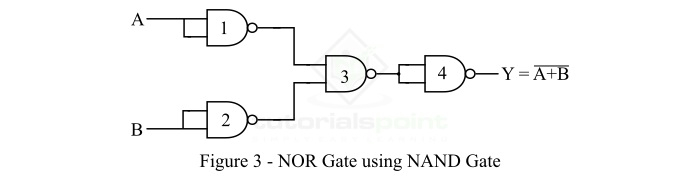# Implementation of NOR Gate from NAND Gate

NOR and NAND gates are universal logic gates, using which we can implement any logic gate or any other logical expression. Read this tutorial to find out how you can implement a NOR gate using a NAND gate.

## What is a NOR Gate?

NOR Gate is a type of universal logic gate, because this logic gate can be used for implementation of any other type of logic gate.

NOR means "NOT + OR". That means the OR output is NOTed or inverted. Therefore, the NOR gate is a combination of OR gate and a NOT gate.

$$\mathrm{NOR\:Gate = OR\:Gate \:+\:NOT\:Gate}$$

A NOR gate is a type of logic gate whose output is HIGH (Logic 1), only when all its inputs are LOW (Logic 0), and it gives an output LOW (Logic 0), even if any of its inputs become HIGH (Logic 1). The logic symbol of a two input NOR gate is shown in Figure-1.### Output Equation of NOR Gate

If A and B are the input variables and Y is the output variable of the NOR gate, then the output of the NOR gate is given by,

$$\mathrm{Y = \overline{A+B} = (A+B)'}$$

It is read as "Y is equal to A plus B whole bar".

### Truth Table of NOR Gate

The table that shows the relationship between inputs and output of a logic gate is referred to as a truth table. The following is the truth table of the NOR gate-

Input

Output

A

B

Y = (A + B)'

0

0

1

0

1

0

1

0

0

1

1

0

## What is a NAND Gate?

The NAND Gate is a type of universal logic gate. Where, a universal logic gate is one that can be used to realize any kind logical expression or any other type of logic gate.

A NAND gate is basically a combination of two basic logic gates namely AND gate and NOT gate, i.e.,

$$\mathrm{NAND\:Logic =AND\:Logic = NOT\:Logic}$$

A NAND gate is the type of logic gate whose output is LOW (Logic 0) when all its inputs are high, and its output is HIGH (Logic 1), when any of its inputs is LOW (Logic 0). Therefore, the operation of the NAND gate is opposite that of the AND gate. The logic symbol of a two input NAND gate is shown in Figure-2.### Output Equation of NAND Gate

If A and B are the input variables and Y is the output variable of the NAND gate, then its output is given by,

$$\mathrm{Y=\overline{A\cdot B} = (A+B)'}$$

It is read as "Y is equal to A.B whole bar".

### Truth Table of NAND Gate

The following is the truth table of the NAND gate-

Input

Output

A

B

Y = (A.B)'

0

0

1

0

1

1

1

0

1

1

1

0

Now, let us discuss the implementation of NOR Gate from NAND Gate.

## Implementation of NOR Gate from NAND Gate

As mentioned above, the NAND gate is a universal logic gate, therefore, it can be used to realize the any other logic gate. The implementation of NOR gate using the NAND gate is shown in Figure-3.From the logic circuit, it is clear that for the implementation of NOR gate using NAND gates only, we require 4 NAND gates. The first two NAND gates perform the complement of input variables A and B, the third NAND gate produce the NAND output of the complemented inputs, i.e. A' and B'. Finally, the fourth NAND gate again operates as an inverter and produce the output Y. This output Y is the equivalent to the output of the NOR gate.

The output of the first and second NAND gates is,

$$\mathrm{Y_1 = \bar{A} \:\:and \:\:Y_2 = \bar{B}}$$

The output of the third NAND gates is,

$$\mathrm{Y_3 = \overline{\bar{A}\cdot \bar{B}} = A+B}$$

The output of the fourth NAND gate is,

$$\mathrm{Y = \overline{A+ B} }$$

Hence, this is the output of a NOR Gate. In this way, we can implement a NOR gate using NAND gates only.

Updated on: 10-Jan-2023

9K+ Views